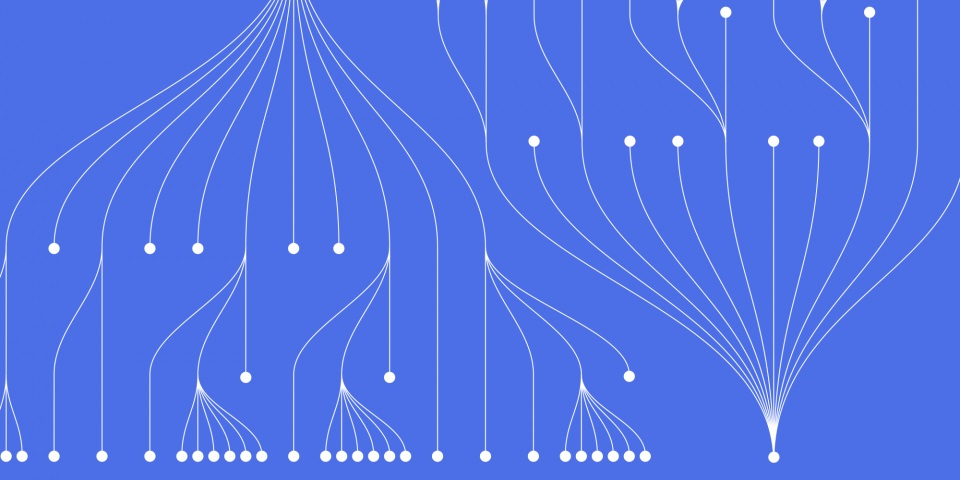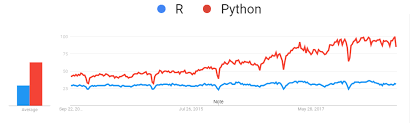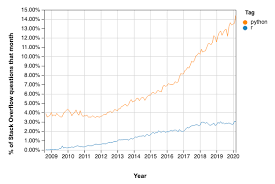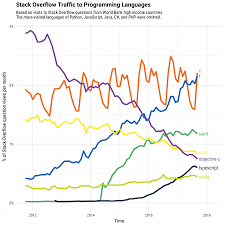23 August 2023

# Machine Learning in R vs Python

Machine learning has transformed fields like computer vision, natural language processing, robotics, and predictive analytics. Mastering machine learning algorithms and techniques is a crucial skill for aspiring data scientists and AI engineers.

The two most popular programming languages for applying machine learning are Python and R. Both languages have robust machine learning libraries and active user communities. But they have some key differences in their ecosystem and approach.

JBI Training offers courses both in Python and R to find out more get in contact or visit our machine learning training courses

In this comprehensive guide, we will compare Python and R for machine learning across various factors. We will look at code examples in both languages. By the end, you should have a clear understanding of the strengths and weaknesses of Python and R for machine learning workloads.

## Popularity for Machine Learning Development

Python has gained tremendous popularity as the preferred language for machine learning development. The chart below illustrates the relative growth in popularity of Python vs R over time for data science and machine learning:Some key reasons that contribute to Python's rising adoption for machine learning include:

• Simplicity and readability - Python's clean and intuitive syntax makes it easy for beginners to learn.
• Extensive machine learning libraries - Python has libraries like Scikit-Learn, TensorFlow, Keras, PyTorch dedicated for machine learning.
• General purpose usage - Python can be used for web development, automation, visualization, and other tasks in addition to machine learning.
• Industry adoption - Leading technology companies have adopted Python for their machine learning systems and operations.
• Strong community - An active ecosystem with forums, blogs, guides that provide ample resources for machine learning practitioners.

R is still widely used in academia for research and statistical modeling. But Python has become the industry standard language for applying machine learning at scale.

## Ease of Learning for Beginners

For programmers who are new to data science and machine learning, Python generally has a shallower learning curve. The syntax of Python is simpler and closer to natural languages like English compared to R.

Let's look at some examples of basic programming constructs and machine learning code in both languages.

#### Variable Assignment

``````
# Python
x = 5
print(x)
```
```
```
```
# R
x <- 5
print(x)
```
```

Python uses standard equals to assignment while R uses the arrow '<-' syntax which can be unfamiliar to beginners.

#### Lists/Vectors

``````
# Python
primes = [2, 3, 5, 7, 11]
print(primes)
```
```
``````
# R
primes <- c(2, 3, 5, 7, 11)
print(primes)
```
```

The square bracket syntax for Python lists is more intuitive compared to using 'c' for combine in R.

#### Linear Regression

``````
# Python
from sklearn.linear_model import LinearRegression

model = LinearRegression()
model.fit(X_train, y_train)
y_pred = model.predict(X_test)
```
```
```
```
# R
model <- lm(y_train ~ X_train)
y_pred <- predict(model, X_test)
```
```

Scikit-learn provides a consistent estimator API while base R requires creating custom model objects.

The R syntax has more operators like '<-' and '~' that beginners have to get accustomed to. Python's coding construct are more standard for programmers from other languages.

## Data Manipulation and Visualization

Both Python and R have mature ecosystems for data manipulation and visualization.

In Python, key packages for data analysis include:

• NumPy - Provides arrays and math operations for numerical data
• Pandas - Offers data frames for managing tabular and time series data
• Matplotlib - Leading plotting and visualization library
• Seaborn - Provides interface for enhanced statistical visualizations

Example of basic data manipulation in Pandas:

``````
import pandas as pd

df = pd.DataFrame({
'Name': ['John', 'Mary', 'Sarah'],
'Age': [25, 32, 18]
})

print(df['Name'])
```
```

In R, the prominent data analysis packages are:

• tidyverse - Collection of packages like dplyr, tidyr for data wrangling
• ggplot2 - Elegant grammar of graphics for visualization
• tibble - Modern reimagining of data frames

Example data wrangling using dplyr:

``````
library(dplyr)

df <- tribble(
~Name, ~Age,
"John", 25,
"Mary", 32,
"Sarah", 18
)

df %>% select(Name)
```
```

Both Python and R provide mature options for preparing, manipulating and exploring data required in machine learning workflows.

## Machine Learning Models and Algorithms

Let's look at some examples of common machine learning algorithms in Python and R.

### Regression

Linear regression can be implemented as:

``````
# Python
from sklearn.linear_model import LinearRegression

model = LinearRegression()
model.fit(X_train, y_train)
y_pred = model.predict(X_test)
```
```
``````
# R
model <- lm(y_train ~ X_train)
y_pred <- predict(model, X_test)
```
```

Here Scikit-learn provides a consistent estimator interface while base R requires creating custom model objects.

### Classification

For classifiers like SVM, the syntax is:

``````
from sklearn.svm import SVC

model = SVC()
model.fit(X_train, y_train)
```
```
``````
library(e1071)

model <- svm(y_train ~ X_train)
```
```

Scikit-learn centralizes common algorithms while R provides them across different packages.

### Clustering

K-means clustering can be implemented as:

``````
from sklearn.cluster import KMeans

model = KMeans(n_clusters=3)
model.fit(X)
```
```
``````
library(stats)

model <- kmeans(X, 3)
```
```

Overall both languages provide access to all the common machine learning algorithms like regressions, SVM, decision trees, k-means, etc. But Python's consistent API for modeling makes it easier to learn.

## Deep Learning Frameworks

For deep learning, Python has gained immense popularity due to its ecosystem of frameworks like TensorFlow, PyTorch and Keras.

Here is an example of a simple neural network in Keras:

``````
from keras.models import Sequential
from keras.layers import Dense

model = Sequential()

model.fit(X_train, y_train, epochs=5)
```
```

In R, deep learning capabilities are provided by packages like Keras, Tensorflow, and MXNet:

```
```
library(keras)

model <- keras_model_sequential()
model %>%
layer_dense(10, input_shape = c(20), activation = 'relu') %>%
layer_dense(1, activation = 'sigmoid')

model %>% compile(
loss = 'binary_crossentropy',
)

model %>% fit(X_train, y_train, epochs = 5)
```
```

The Python APIs feel more native while R relies on wrappers around the other frameworks.

Overall, Python provides a richer ecosystem of production-ready frameworks for deep learning like PyTorch, TensorFlow and Keras.

## Deploying Machine Learning Models

Python provides a smoother path for taking machine learning models into production environments.

Frameworks like Flask and Django allow wrapping Python models into web APIs with just a few lines of code:

``````
import pickle

@app.route('/predict', methods=['POST'])
def infer():

# Extract features from request
features = request.form[[]]

# Load model and make prediction
with open('model.pkl', 'rb') as f:

pred = model.predict([features])
return str(pred)

if __name__=='__main__':
app.run(debug=True, host='0.0.0.0')
```
```

Python also offers streamlined deployment options on major cloud platforms like AWS, GCP and Azure.

In R, more custom engineering is required to wrap models into production APIs. IDEs like RStudio allow creating basic APIs using Plumber or Shiny. But large scale deployment involves additional tooling.

So for taking models into live production systems, Python offers a smoother path compared to R.

## Scalability for Large Datasets

Python along with its computational libraries provides powerful alternatives for scaling up machine learning:

• Numba gives access to GPU acceleration from Python for performance gains.
• Dask allows scaling Python workflows across clusters to handle big data.
• TensorFlow Distributed enables easy distributed training of deep learning models.

Here is an example using Dask to train a model in parallel:

``````
from sklearn.ensemble import RandomForestClassifier

# Fit model across multiple workers
model = RandomForestClassifier()
```
```

In R, parallel computing options are available through add-on packages:

• foreach provides iterative execution
• doParallel enables parallel backends
• rddtools integrates with Spark for big data

But Python provides greater flexibility for scaling computation with its multiprocess, GPU and cluster computing libraries.

## Community Support

Both Python and R enjoy active open source ecosystems with forums, blogs, guides and Q&A sites that offer learning resources.

Some popular communities for machine learning practitioners include:

• Stack Overflow - Key site for technical questions and answers
• Reddit - Discussion forums like r/learnpython, r/learnmachinelearning
• Kaggle - Platform for datasets, competitions and community

However, Python's adoption has created a much larger community with content dedicated specially to data science and machine learning.

The immense popularity means beginners can find answers and help more easily for Python related questions on communities like Stack Overflow.The wider resources, guides and tutorials around Python machine learning give it an edge for beginners entering the field.

## Usage in Industry

Today usage of Python dominates over R for machine learning in the industry. This includes both tech giants and startups applying machine learning.

Nearly all the major technology firms like Google, Facebook, Microsoft, etc. use Python-based stacks for their machine learning systems and production workloads.

Python's versatility, scalability and deployment capabilities have made it the language of choice for implementing machine learning engineering in practice. R is still used in many companies but more for statistical modeling and analysis.

Here is a breakdown of Python vs R usage for machine learning based on a Kaggle survey of data professionals:Python is the clear leader for applying machine learning engineering across domains like computer vision, natural language processing, forecasting, robotics, and more.

## Summary

To recap, here are some key points comparing R and Python for machine learning:

• Python is more beginner friendly with its intuitive syntax and coding constructs. R has a steeper learning curve.
• Both provide extensive libraries for data manipulation, visualization and modeling. But Python's consistent API makes it easier to learn.
• For deep learning, Python provides more frameworks like PyTorch, TensorFlow and Keras. R has wrappers to access these libraries.
• Python offers smoother deployment of models into production and integration with web apps.
• Python provides greater scalability through its distributed computing libraries.
• Python has a much larger community with abundant tutorials, guides and help dedicated specially to machine learning.

While both languages are capable for machine learning, Python stands out for its ease of use and capabilities for applying machine learning at scale. For delivering production grade machine learning systems, Python offers some clear advantages over R.

If you have enjoyed this article please feel free to browse our blog for more useful articles and guides. You may enjoy python machine learning FAQs questions or how to build machine learning model pythonTech Blogger & Researcher for JBI Training

CONTACT
+44 (0)20 8446 7555

[email protected]

SHAREJB International Training Ltd  -  Company Registration Number: 08458005
Registered Address: Wohl Enterprise Hub, 2B Redbourne Avenue, London, N3 2BS

POPULAR